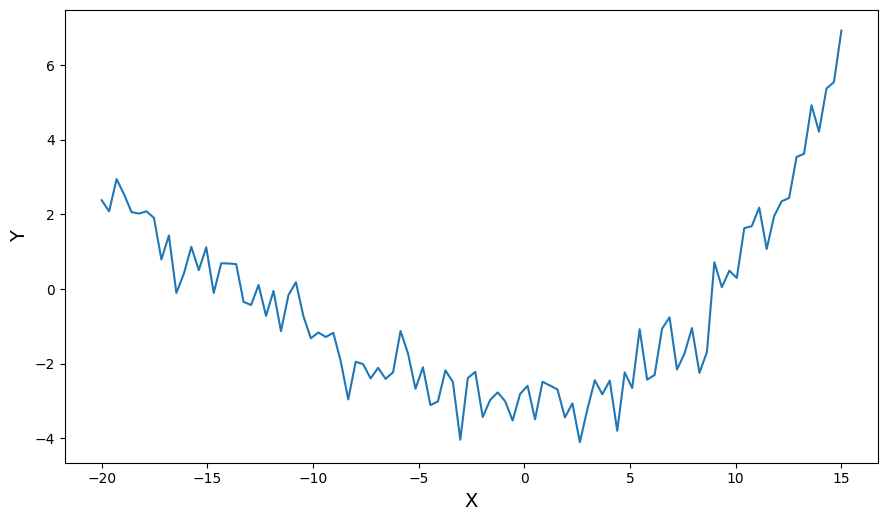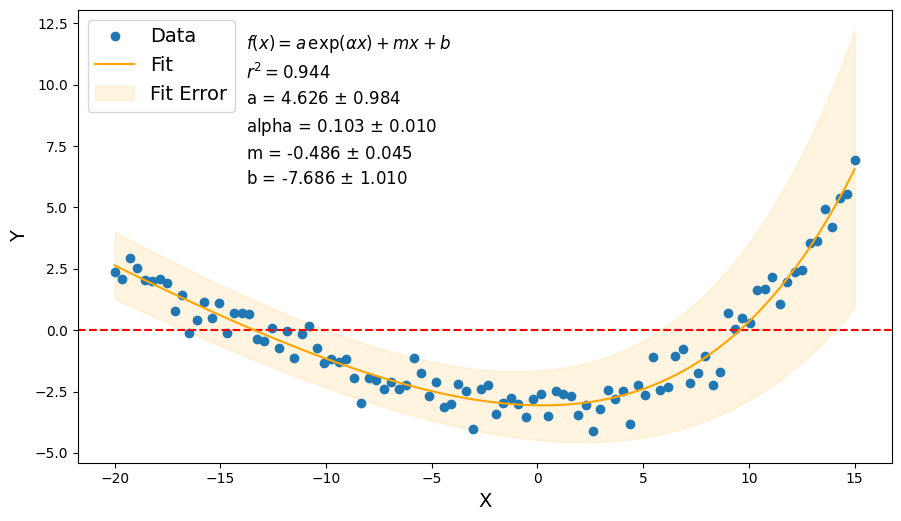# Fit Functions¶

Fit functions are a set of callable classes designed to aid in fitting analytical functions to data. A fit function class combines the following functionality:

1. An analytical function that is callable with given parameters or fitted parameters.

2. Curve fitting functionality (usually SciPy’s curve_fit() or linregress()), which stores the fit statistics and parameters into the class. This makes the function easily callable with the fitted parameters.

3. Error propagation calculations.

4. A root solver that returns either the known analytical solutions or uses SciPy’s fsolve() to calculate the roots.

:

%matplotlib inline

import matplotlib.pyplot as plt
import numpy as np

from pathlib import Path

from plasmapy.analysis import fit_functions as ffuncs

plt.rcParams["figure.figsize"] = [10.5, 0.56 * 10.5]


## Fit function basics¶

There is an ever expanding collection of fit functions, but this notebook will use ExponentialPlusLinear as an example.

A fit function class has no required arguments at time of instantiation.

:

# basic instantiation
explin = ffuncs.ExponentialPlusLinear()

# fit parameters are not set yet
(explin.params, explin.param_errors)

:

(None, None)


Each fit parameter is given a name.

:

explin.param_names

:

('a', 'alpha', 'm', 'b')


These names are used throughout the fit function’s documentation, as well as in its __repr__, __str__, and latex_str methods.

:

(explin, explin.__str__(), explin.latex_str)

:

(f(x) = a exp(alpha x) + m x + b <class 'plasmapy.analysis.fit_functions.ExponentialPlusLinear'>,
'f(x) = a exp(alpha x) + m x + b',
'a \\, \\exp(\\alpha x) + m x + b')


## Fitting to data¶

Fit functions provide the curve_fit() method to fit the analytical function to a set of $$(x, y)$$ data. This is typically done with SciPy’s curve_fit() function, but fitting is done with SciPy’s linregress() for the Linear fit funciton.

Let’s generate some noisy data to fit to…

:

params = (5.0, 0.1, -0.5, -8.0)  # (a, alpha, m, b)
xdata = np.linspace(-20, 15, num=100)
ydata = explin.func(xdata, *params) + np.random.normal(0.0, 0.6, xdata.size)

plt.plot(xdata, ydata)
plt.xlabel("X", fontsize=14)
plt.ylabel("Y", fontsize=14)

:

Text(0, 0.5, 'Y')The fit function curve_fit() shares the same signature as SciPy’s curve_fit(), so any **kwargs will be passed on. By default, only the $$(x, y)$$ values are needed.

:

explin.curve_fit(xdata, ydata)


### Getting fit results¶

After fitting, the fitted parameters, uncertainties, and coefficient of determination, or $$r^2$$, values can be retrieved through their respective properties, params, parame_errors, and rsq.

:

(explin.params, explin.params.a, explin.params.alpha)

:

(FitParamTuple(a=6.386777927216642, alpha=0.0881570433129568, m=-0.5393527238720933, b=-9.308409800168333),
6.386777927216642,
0.0881570433129568)

:

(explin.param_errors, explin.param_errors.a, explin.param_errors.alpha)

:

(FitParamTuple(a=1.4565869852537874, alpha=0.009192101779287832, m=0.05799525216190728, b=1.4743736063896702),
1.4565869852537874,
0.009192101779287832)

:

explin.rsq

:

0.9451608237258722


### Fit function is callable¶

Now that parameters are set, the fit function is callable.

:

explin(0)

:

-2.921631872951691


Associated errors can also be generated.

:

y, y_err = explin(np.linspace(-1, 1, num=10), reterr=True)
(y, y_err)

:

(array([-2.92121416, -2.9253789 , -2.92725484, -2.92679672, -2.92395834,
-2.91869262, -2.91095153, -2.90068612, -2.88784643, -2.87238154]),
array([1.98965372, 2.00683718, 2.02471224, 2.04329621, 2.06260682,
2.08266221, 2.10348094, 2.12508197, 2.14748473, 2.17070908]))


Known uncertainties in $$x$$ can be specified too.

:

y, y_err = explin(np.linspace(-1, 1, num=10), reterr=True, x_err=0.1)
(y, y_err)

:

(array([-2.92121416, -2.9253789 , -2.92725484, -2.92679672, -2.92395834,
-2.91869262, -2.91095153, -2.90068612, -2.88784643, -2.87238154]),
array([1.98965514, 2.00683765, 2.02471227, 2.04329634, 2.06260763,
2.08266426, 2.10348483, 2.12508832, 2.14749416, 2.17072224]))


### Plotting results¶

:

# plot original data
plt.plot(xdata, ydata, marker="o", linestyle=" ", label="Data")
ax = plt.gca()
ax.set_xlabel("X", fontsize=14)
ax.set_ylabel("Y", fontsize=14)

ax.axhline(0.0, color="r", linestyle="--")

# plot fitted curve + error
yfit, yfit_err = explin(xdata, reterr=True)
ax.plot(xdata, yfit, color="orange", label="Fit")
ax.fill_between(
xdata,
yfit + yfit_err,
yfit - yfit_err,
color="orange",
alpha=0.12,
zorder=0,
label="Fit Error",
)

# plot annotations
plt.legend(fontsize=14, loc="upper left")

txt = f"$f(x) = {explin.latex_str}$\n" f"$r^2 = {explin.rsq:.3f}$\n"
for name, param, err in zip(explin.param_names, explin.params, explin.param_errors):
txt += f"{name} = {param:.3f} $\\pm$ {err:.3f}\n"
txt_loc = [-13.0, ax.get_ylim()]
txt_loc = ax.transAxes.inverted().transform(ax.transData.transform(txt_loc))
txt_loc -= 0.02
txt_loc -= 0.05
ax.text(
txt_loc,
txt_loc,
txt,
fontsize="large",
transform=ax.transAxes,
va="top",
linespacing=1.5,
)

:

Text(0.2072727272727273, 0.9500000000000002, '$f(x) = a \\, \\exp(\\alpha x) + m x + b$\n$r^2 = 0.945$\na = 6.387 $\\pm$ 1.457\nalpha = 0.088 $\\pm$ 0.009\nm = -0.539 $\\pm$ 0.058\nb = -9.308 $\\pm$ 1.474\n')### Root solving¶

An exponential plus a linear offset has no analytical solutions for its roots, except for a few specific cases. To get around this, ExponentialPlusLinear().root_solve() uses SciPy’s fsolve() to calculate it’s roots. If a fit function has analytical solutions to its roots (e.g. Linear().root_solve()), then the method is overriden with the known solution.

:

root, err = explin.root_solve(-15.0)
(root, err)

:

(-13.728200661485241, nan)


Let’s use Linear().root_solve() as an example for a known solution.

:

lin = ffuncs.Linear(params=(1.0, -5.0), param_errors=(0.1, 0.1))
root, err = lin.root_solve()
(root, err)

:

(5.0, 0.5099019513592785)